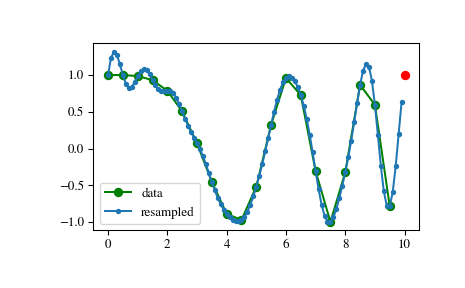# scipy.signal.resample¶

scipy.signal.resample(x, num, t=None, axis=0, window=None)[source]

Resample x to num samples using Fourier method along the given axis.

The resampled signal starts at the same value as x but is sampled with a spacing of len(x) / num * (spacing of x). Because a Fourier method is used, the signal is assumed to be periodic.

Parameters: x : array_like The data to be resampled. num : int The number of samples in the resampled signal. t : array_like, optional If t is given, it is assumed to be the sample positions associated with the signal data in x. axis : int, optional The axis of x that is resampled. Default is 0. window : array_like, callable, string, float, or tuple, optional Specifies the window applied to the signal in the Fourier domain. See below for details. resampled_x or (resampled_x, resampled_t) Either the resampled array, or, if t was given, a tuple containing the resampled array and the corresponding resampled positions.

decimate
Downsample the signal after applying an FIR or IIR filter.
resample_poly
Resample using polyphase filtering and an FIR filter.

Notes

The argument window controls a Fourier-domain window that tapers the Fourier spectrum before zero-padding to alleviate ringing in the resampled values for sampled signals you didn’t intend to be interpreted as band-limited.

If window is a function, then it is called with a vector of inputs indicating the frequency bins (i.e. fftfreq(x.shape[axis]) ).

If window is an array of the same length as x.shape[axis] it is assumed to be the window to be applied directly in the Fourier domain (with dc and low-frequency first).

For any other type of window, the function scipy.signal.get_window is called to generate the window.

The first sample of the returned vector is the same as the first sample of the input vector. The spacing between samples is changed from dx to dx * len(x) / num.

If t is not None, then it represents the old sample positions, and the new sample positions will be returned as well as the new samples.

As noted, resample uses FFT transformations, which can be very slow if the number of input or output samples is large and prime; see scipy.fftpack.fft.

Examples

Note that the end of the resampled data rises to meet the first sample of the next cycle:

>>> from scipy import signal

>>> x = np.linspace(0, 10, 20, endpoint=False)
>>> y = np.cos(-x**2/6.0)
>>> f = signal.resample(y, 100)
>>> xnew = np.linspace(0, 10, 100, endpoint=False)

>>> import matplotlib.pyplot as plt
>>> plt.plot(x, y, 'go-', xnew, f, '.-', 10, y, 'ro')
>>> plt.legend(['data', 'resampled'], loc='best')
>>> plt.show()#### Previous topic

scipy.signal.detrend

#### Next topic

scipy.signal.resample_poly